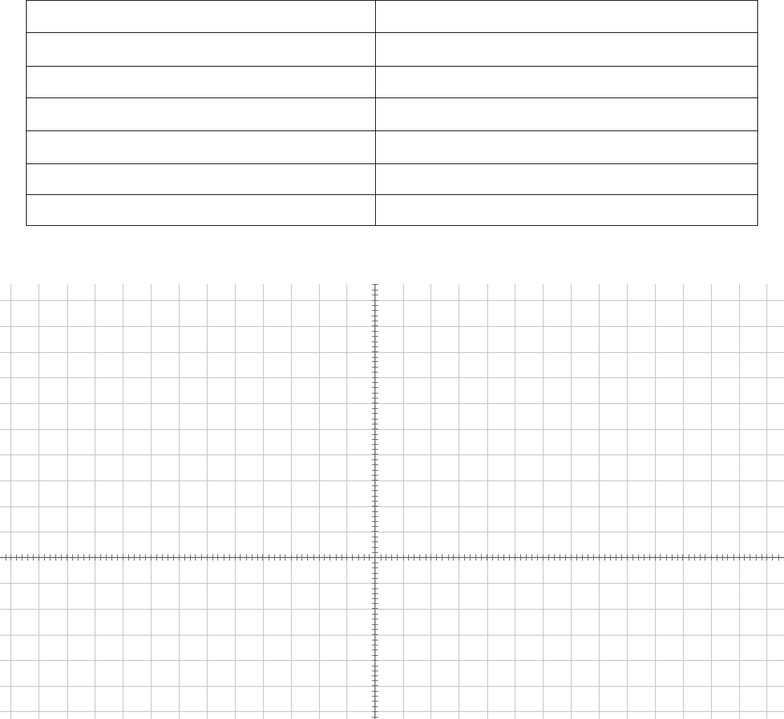Study Guides (380,000)
US (220,000)
ISU (1,000)
MAT (200)
MAT 145 (70)
All (70)
Midterm

# MATH 145 Illinois State Test03part02S15Exam

Department
Mathematics
Course Code
MAT 145
Professor
All
Study Guide
Midterm

This preview shows page 1. to view the full 5 pages of the document.MAT 145: Test #3 (50 points)
Part 2: Calculator OK!
Name ________________________ Calculator Used ____________ Score ____________________
21. For
f(x)=8x3+81x242x8
, defined for all real numbers, use calculus techniques to determine all
intervals on which f is increasing and on which f is decreasing. Show all calculations. Explain your
answer, using calculus, in a sentence. (2 pts)
Increasing on: _______________________
(intervals: exact values)
Decreasing on: _______________________
(intervals: exact values)
22. Use calculus techniques to determine the location of any local maxima and any local minima of
f(x)=8x3+81x242x8
, where f is defined for all real numbers. Show all calculations. Explain your
answer, using calculus, in a sentence. (2 pts)
Local Maxima at: ____________________
(exact x values)
Local Minima at: _____________________
(exact x values)
23. For
g(x)=x33x2+x2
, defined for all real numbers, use calculus techniques to determine all
intervals on which g is concave up and on which g is concave down. Show all calculations. Explain your
answer, using calculus, in a sentence. (2 pts)
Concave Up on: ______________________
(intervals: exact values)
Concave Down on: ___________________
(intervals: exact values)
24. Use calculus techniques to determine ordered pairs for any points of inflection of
g(x)=x33x2+x2
,
where g is defined for all real numbers. Show all calculations. Explain your answer, using calculus, in a
sentence. (2 pts)
Points of Inflection: ___________________
(ordered pairs: exact values)
###### You're Reading a Preview

Unlock to view full version

Only page 1 are available for preview. Some parts have been intentionally blurred.25. Use the information here to sketch the graph of a function f that meets ALL the following requirements.
Label your graph to help me identify these requirements. (12 pts)
lim
x→−∞ f(x)=2
lim
xf(x)=0
lim
x2+f(x)=
There is a local minimum at x = –2.
There is an absolute minimum at x = 5.
!
f(x)>0
on
2,0
()
0,2
()
5,
()
!
f(x)<0
on
−∞,2
()
2,5
()
!!
f(x)>0
on
4,1
()
0,2
()
(2,6)
!!
f(x)<0
on
−∞,4
()
∪ −1,0
()
6,
()
The y-intercept is (0, 2).
Exactly two x-intercepts: (–2, 0) and (3, 0)
A point on the graph is (–4, 1).
A point on the graph is (5, –2).
5
4.5
4
3.5
3
2.5
2
1.5
1
0.5
0.5
– 1
1.5
– 2
2.5
– 3
– 6 – 5 – 4 – 3 – 2 – 1 1 2 3 4 5 6 7
###### You're Reading a Preview

Unlock to view full version